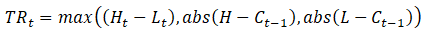The average true range or ATR for short is a way of measuring volatility in price. One useful aspect of the ATR is that it captures both intraday volatility and between day volatility. Other measures based on standard deviation of prices usually only do one or the other.

J. Welles Wilder Jr. created the ATR for futures trading but its use in technical analysis is now fairly widespread.

This article looks at the ATR calculations, as well as the uses that ATR has in trading and risk management.

## The ATR Formula

To calculate the ATR we first need to find the true range at a point in time. The true range (TR) comes from the formula:The True Range at a point t is the greater of:

1. Current period’s price range ( Ht – Lt)
2. Current high minus previous close
3. Current low minus previous close

The first metric captures the amount of intraday volatility. The second metric captures the amount that the price has gapped up between the two periods. The third captures the amount the price has gapped down between the periods.

The value for the ATR at time t is then the moving average of the true range (TR). The period of the moving average then determines the scale of the ATR. For example, ATR-14 deploys a 14 point moving average.

One of the key differences between ATR and other volatility metrics is that ATR uses price ranges rather than price percentage changes or price log ratios.

This means ATR is not absolute but is dependent on price. Financial instruments with higher prices will have higher ATR than those with lower prices for the same percentage of price change.

## ATR vs Standard Deviation

While at first glance ATR and standard deviation (SD) may seem similar, there are some subtle differences. Knowing these will help to decide which metric is appropriate.

The chart in Figure 1 shows how ATR and standard deviation compare to one another. The red line is the ATR and the green is standard deviation, both with period 14.

The first main discrepancy is at (1) and (2) where ATR is falling but the SD is rising. The SD increases here where the trend is falling because of the changing price levels with respect to the moving average. By contrast the ATR does not respond, and even falls because the intraday and between day-price changes are small.

The second discrepancy is at (3) and (4) where the ATR rises but the SD is falling. The SD is falling because the price reversal is causing it to return towards its mean value over the 14 point moving average. On the other hand the ATR rises, because the intraday volatility is rising as we see from the increasing candle sizes.

For the above reasons, it can be helpful to use the ADX as well as the ATR because ADX measures trend.

## ATR, Risk and Money Management

ATR is a measure of price range volatility but it is also a measure of risk. The ATR of an instrument is helpful in a few different scenarios.  In a trading system, it can help in deciding position sizing. It can also help in setting your stop loss and take profit.

READ ALSO  Jim Bianco – Global Macro Series – 21st August 2020

The ATR helps you to do some basic risk management. The turtle strategy uses exactly this method.

Take EUR/USD as an example. The ATR-14 for EUR/USD is presently 0.0084. Suppose we want to trade a contract size of \$10,000. Start by computing the daily range.

Daily range \$ = 0.0084 × \$10,000 =  \$84

The ATR is telling us to expect a range of daily movements of plus or minus \$84 in the position value.

This information is a great help in money management. For example if we apply a 1 percent risk-rule, we can adjust the position sizes so that the daily range never exceeds 1% of the account value.

If our account’s equity were \$5000, we know we could only safely trade ½ of one contract to stay within the risk limit. A trade of this size would have a daily range of \$42 and that is easily inside the 1 percent risk limit.

## ATR and Stop Losses

The second application for ATR is in calculation of stop losses. In an equal amount of time there’s a greater chance of a stop loss being breached when ATR is high than when it is low.

That ATR measures both intra period and between period volatility makes it a useful tool in this respect. For example, a standard deviation of closing prices will not respond at all to ranging that is going on within each period whereas the ATR will. A standard deviation could give an unduly low reading for volatility and lead to placing stop losses too close.

One limitation of ATR in setting stop losses and take profit is that it may not respond to trending as Figure 1 demonstrates. For that reason, it is necessary to use ATR alongside other methods.

The ATR is useful in confirming the readings of other indicators. One such application is with the Bollinger bands. In this case, the ATR can help detect and trade volatility squeezes.

Volatility squeezes are places in which the price becomes restricted within a tight range before breaking out suddenly and often explosively. Naturally, traders want to identify these events because they can make for very profitable trading opportunities.

Figure 2 shows a volatility squeeze taking place and its subsequent breakout.

Here the ATR helps to confirm the actual price action that is taking place. Just prior to the breakout, we see a narrowing of the Bollinger bands (falling standard deviation). Meanwhile the ATR drops to an extreme low. This happens as we see a compression of the candle lengths as the squeeze finalizes.

The squeeze ends with a powerful bullish breakout.